```!pip install -Uqq fastbook
import fastbook
fastbook.setup_book()
```

```%matplotlib inline

from random import shuffle
from fastbook import *
from kaggle import api
from pandas.api.types import is_string_dtype, is_numeric_dtype, is_categorical_dtype
from fastai.tabular.all import *
from sklearn.ensemble import RandomForestRegressor, RandomForestClassifier
from sklearn.tree import DecisionTreeRegressor, DecisionTreeClassifier, export_graphviz
from dtreeviz.trees import *
from IPython.display import Image, display_svg, SVG

import pandas as pd

pd.options.display.max_rows = 20
pd.options.display.max_columns = 8
```

# Introduction

## Objective

The objective is to model the KDD'99 dataset an anomaly detection dataset.

## Dataset

KDD'99 is a typical old school dataset. One surprising aspect was that there were no timestamps or system identifiers. This made it difficult to see how to associate the rows with each other in any meaningul way. The task is to assume that each observation is independent and identically distributed.

The dataset contains 31 features including the target

This study leaves for future an evaluation of whether the kaggle KDD99 dataset is the same as the original KDD99 dataset.

## Benchmarks on KDD'99 (Current SOA: marked with *)

Papers with code shows the expected outcome metrics for a variety of learning methods.

## Findings

• RF and Ensemble performs best according to the paper.
• Sklearn implements CART and it beats all the benchmarks in the paper.

This notebook is a Frankenstien creation from me adapting the FastAI Tabular Data Toolset, 2020 to a diferent dataset than was used in the tutorial. The significant differences between my notebook and the FastAI tutorial are:

• Adaptation of FastAI to KDD'99
• Example of using FastAI 2 for a canonical classification problem
• The deep dive into contributions profiling found here. This will be encorporated into the prcvd.ai core library.
• Removal of toy/tutorial content from the FastAI such as the look into OOB errors and the discussion of neural networks for continuous features.

# Code

## Dataset configuration

We sourced the dataset by downloading it manually from kaggle. The configuration parameters below were also extracted manually from the accompanying kaggle description. Of particular interest was to verify the categorical and numerical features separately.

```data_dir = Path('/Users/home0/data/anomaly-detect/archive/')
train_ds = data_dir/'Train.txt'

cat_str_names = ['protocol_type', 'service', 'flag']
cat_names = bin_names + cat_str_names
num_names = ['duration','src_bytes','dst_bytes','wrong_fragment',
'num_root','num_file_creations','num_shells',
'num_access_files','num_outbound_cmds','count',
'srv_count','serror_rate','srv_serror_rate',
'rerror_rate','srv_rerror_rate','same_srv_rate',
'diff_srv_rate','srv_diff_host_rate','dst_host_count',
'dst_host_srv_count','dst_host_same_srv_rate',
'dst_host_diff_srv_rate','dst_host_same_src_port_rate',
'dst_host_serror_rate','dst_host_srv_serror_rate',
'dst_host_rerror_rate','dst_host_srv_rerror_rate']
procs = [Categorify, FillMissing, Normalize]
dep_var = 'attack'
"duration","protocol_type","service","flag","src_bytes",
"dst_bytes","land",
"num_compromised","root_shell","su_attempted","num_root",
"num_file_creations",
"num_shells","num_access_files","num_outbound_cmds",
"srv_serror_rate",
"rerror_rate","srv_rerror_rate","same_srv_rate", "diff_srv_rate",
"srv_diff_host_rate","dst_host_count","dst_host_srv_count",
"dst_host_same_srv_rate",
"dst_host_diff_srv_rate","dst_host_same_src_port_rate",
"dst_host_srv_diff_host_rate","dst_host_serror_rate","dst_host_srv_serror_rate",
"dst_host_rerror_rate","dst_host_srv_rerror_rate","attack",
"last_flag"]
```

```df = pd.read_csv(train_ds, header=None)
```

### Looking at unique values in each column

Even though the dataset specification provided by the creators of KDD'99 specify that some features are numerical, they don't have the same variation that one might expect with true continuous features. Henceforth, we treat those features with a numerical type as continuous features while the boolean or string type fields are treated as categoricals. As we know, a truly continously distributed random variable will theoretically never be the same. Some common reasons why numerical features are not truly continuous are:

• the device sampling has low sensitivity
• the ETL/ELT processes between the measurement device and us are losing significant digits.

This is helpful to understand because it stands to reason that some types of attacks will be only visible if the data is highly expressive.

```print('Dataset Shape', df.shape)
[(col,len(df[col].drop_duplicates()))for col in df.columns]
```
```Dataset Shape (125973, 43)
```
```[('duration', 2981),
('protocol_type', 3),
('service', 70),
('flag', 11),
('src_bytes', 3341),
('dst_bytes', 9326),
('land', 2),
('wrong_fragment', 3),
('urgent', 4),
('hot', 28),
('logged_in', 2),
('num_compromised', 88),
('root_shell', 2),
('su_attempted', 3),
('num_root', 82),
('num_file_creations', 35),
('num_shells', 3),
('num_access_files', 10),
('num_outbound_cmds', 1),
('count', 512),
('srv_count', 509),
('serror_rate', 89),
('srv_serror_rate', 86),
('rerror_rate', 82),
('srv_rerror_rate', 62),
('same_srv_rate', 101),
('diff_srv_rate', 95),
('srv_diff_host_rate', 60),
('dst_host_count', 256),
('dst_host_srv_count', 256),
('dst_host_same_srv_rate', 101),
('dst_host_diff_srv_rate', 101),
('dst_host_same_src_port_rate', 101),
('dst_host_srv_diff_host_rate', 75),
('dst_host_serror_rate', 101),
('dst_host_srv_serror_rate', 100),
('dst_host_rerror_rate', 101),
('dst_host_srv_rerror_rate', 101),
('attack', 23),
('last_flag', 22)]```

## Training / Testing Splits

Unlike many tabular datasets that have primary keys to distinguish rows from each other, this dataset has none. That is almost certainly because the dataset has been anonymized and scrubbed. There is nothing we we care to do about that, assuming that the dataset has been anonymized correctly. So, for the training - testing split, we simply take a random selection of rows. Like most of my experiments, the `train_perc` (the percentage of the dataset used in training, where `1-train_perc` is the percentage of the dataset used for OOB testing) is a hyperparameter in the sense that we may choose to vary it and see how that effects the modeling outcomes.

```from random import shuffle
shuf = list(df.index)
shuffle(shuf)
train_perc = 0.90
train_ct = int(len(shuf)*train_perc)
splits = (shuf[:train_ct], shuf[train_ct:])
cont = num_names
cat = cat_names
```

```procs = [Categorify, FillMissing]
to = TabularPandas(df, procs, cat, cont, y_names=dep_var, splits=splits)
xs, y = to.train.xs,to.train.y
valid_xs,valid_y = to.valid.xs,to.valid.y
```

## Keeping Track of Meaning with Categorical Targets

The datablock API will want to convert all categorical variables to integers, but when we go to try to understand and explain how the model is working, those meanings get lost. The object `name_to_id` is used to keep track of the mapping between the way the models see the data and the way we understand the data. Potentially, the most important detail I learned in this exercise was how the FastAI datablock API keeps track of the mapping between categorical target names (the language strings that describe your classes) and the numeric (`int`) representation that is used by the models. This will become important later when we start trying to understand how each column contributes to the prediction. This is not covered in the FastAI tutorial on tabular data because in that case, they examine regression models which have continuous targets. In this case, we have a categorical target.

```name_to_id = {name: i for i,name in enumerate(to.categorize.vocab)}
name_to_id
```
```{'back': 0,
'buffer_overflow': 1,
'ftp_write': 2,
'guess_passwd': 3,
'imap': 4,
'ipsweep': 5,
'land': 6,
'multihop': 8,
'neptune': 9,
'nmap': 10,
'normal': 11,
'perl': 12,
'phf': 13,
'pod': 14,
'portsweep': 15,
'rootkit': 16,
'satan': 17,
'smurf': 18,
'spy': 19,
'teardrop': 20,
'warezclient': 21,
'warezmaster': 22}```

### Target Names

It is not clear to me at the time of writing this that this is the correct way to map the target class names to the ids created by the Data Block API. However, it is my best guess. Link to forum topic here

## First model - Simple and Easy to Introspect

The first model is a toy model so we can examin the model and do some error checking. Here, we have a smell check to see that the first model fits some semblance of intuition we have from common sense.

```m = DecisionTreeClassifier(max_leaf_nodes=4)
m.fit(xs, y);
```

```draw_tree(m, xs, size=14, leaves_parallel=True, precision=2)
```
<!DOCTYPE svg PUBLIC "-//W3C//DTD SVG 1.1//EN" "http://www.w3.org/Graphics/SVG/1.1/DTD/svg11.dtd">

## Success Metrics / Loss / Error Estimation

Here, we're looking at the extent to which we classify correctly compared to the total observations in the test set. Note, they are not root mean squared error. I left the functions the same name as in the fastai tutorial for easy of copy/pasting code between notebooks.

```def r_mse(pred, y):
out = sum(np.equal(pred, y)) / len(y)
return out
def m_rmse(m, xs, y): return r_mse(m.predict(xs), y)
```

## Train a full model (single CART tree)

Train a single CART Tree with default parameters (See:`from sklearn.tree import DecisionTreeClassifier`). Benchmarks did not evaluate CART, but it is very similar in its approach to the C4.5 tree evaluated in the benchmarking paper.

```m = DecisionTreeClassifier()
m.fit(xs, y);
```

```m_rmse(m, xs, y.values), m_rmse(m, valid_xs, valid_y)
```
`(0.9990826901874311, 0.99190347674234)`

This is extremely high model performance from a single tree. It already exceeds the state of the art that is from the Papers With Code Article, although in the same ballpark.

## Train a Random Forest with 4 parameters

The model performs really well. Even if we constrain it to having no singleton leaves. The reason we might want to increase `MIN_SAMPLES_PER_LEAF` is that we associate stability or generalizability of the solution with the number of leaves. If we have only leaves that have multiple instances in the training set, then we expect those are repeatable out of training sample. Even constraining each leaf to need at least 50 instances we see that the model accurately classifies >99% of the testing instances.

```N_ESTIMATORS=40
MAX_SAMPLES=100_000
MAX_FEATURES=10
MIN_SAMPLES_LEAF=150

def rf(xs, y,
n_estimators=N_ESTIMATORS,
max_samples=MAX_SAMPLES,
max_features=MAX_FEATURES,
min_samples_leaf=MIN_SAMPLES_LEAF,
**kwargs):
model = RandomForestClassifier(
n_jobs=-1,
n_estimators=n_estimators,
max_samples=max_samples,
max_features=max_features,
min_samples_leaf=min_samples_leaf,
oob_score=True)
return model.fit(xs, y)
```

```MIN_SAMPLES_LEAFS=[1, 10, 100, 200, 400, 800, 1000]
for ms in MIN_SAMPLES_LEAFS:
m = rf(xs, y, min_samples_leaf=ms);

print(
'min_samples_per_leaf={}, train error={}, valid error={}'
.format(ms,m_rmse(m, xs, y),m_rmse(m, valid_xs, valid_y))
)
```
```min_samples_per_leaf=1, train error=0.9989768467475193, valid error=0.9934910303222734
min_samples_per_leaf=10, train error=0.9939316427783903, valid error=0.9932528972852834
min_samples_per_leaf=100, train error=0.9896626240352812, valid error=0.9900777901254167
min_samples_per_leaf=200, train error=0.9830385887541345, valid error=0.9829337990157168
min_samples_per_leaf=400, train error=0.973406835722161, valid error=0.9732497221781236
min_samples_per_leaf=800, train error=0.9568158765159868, valid error=0.955072233687887
min_samples_per_leaf=1000, train error=0.9506152149944873, valid error=0.9477694872201937
```

```preds = np.stack([t.predict(valid_xs) for t in m.estimators_])
preds_std = preds.std(0)
```

```plt.plot([r_mse(preds[:i+1].mean(0), valid_y) for i in range(100)]);
```## Feature Importance from trees in the Random Forest

Feature importance measures the effectiveness of the split chosen by the CART algorithm based on the data.

Feature importance is calculated as the decrease in node impurity weighted by the probability of reaching that node. The node probability can be calculated by the number of samples that reach the node, divided by the total number of samples. The higher the value the more important the feature.

Stacey Ronaghan - paywalled Below, we examine the feature importance using the various approaches proposed in the FastAI course. Below is a

```def rf_feat_importance(m, df):
return pd.DataFrame({'cols':df.columns, 'imp':m.feature_importances_}
).sort_values('imp', ascending=False)
```

```fi = rf_feat_importance(m, xs)
fi[:10]
```
cols imp
28 same_srv_rate 0.231520
10 src_bytes 0.133203
36 dst_host_serror_rate 0.101403
29 diff_srv_rate 0.092543
37 dst_host_srv_serror_rate 0.070134
8 flag 0.069167
6 protocol_type 0.040768
11 dst_bytes 0.034322
25 srv_serror_rate 0.028164
35 dst_host_same_src_port_rate 0.025868

```def plot_fi(fi):
return fi.plot('cols', 'imp', 'barh', figsize=(12,7), legend=False)

plot_fi(fi[:30]);
```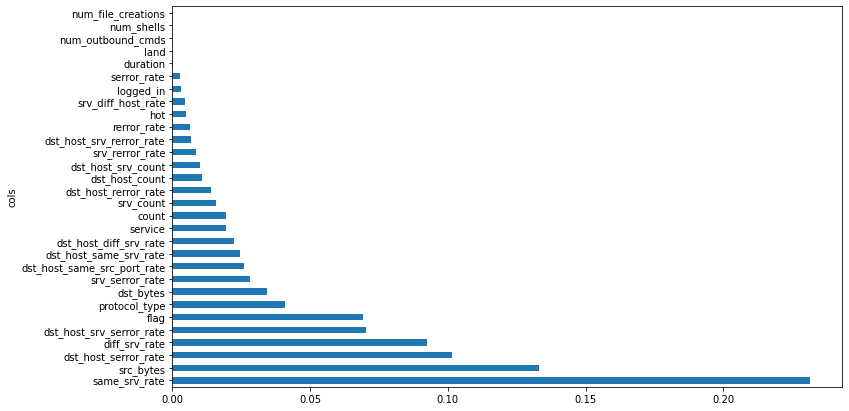### Removing Features

Using feature importance, it is possible to discover which features can be removed without significant loss of model effectiveness. Using feature importance to assign priority to each feature, we can selectively remove the low importance features individually and retrain the model to estimate the impact.

```to_keep = fi[fi.imp>0.005].cols
len(to_keep)
```
`21`

```xs_imp = xs[to_keep]
valid_xs_imp = valid_xs[to_keep]
```

```m = rf(xs_imp, y)
```

```m1 = rf(xs, y, min_samples_leaf=10)
m2 = rf(xs_imp, y, min_samples_leaf=10)
print(
'Original feature set\nfeature_count={}, train error={:.4f}, valid error={:.4f}'
.format(len(xs.columns),
m_rmse(m1, xs, y),
m_rmse(m1, valid_xs, valid_y)
)
)

print(
'Reduced feature set\nfeature_count={}, train error={:.4f}, valid error={:.4f}'
.format(len(xs_imp.columns),
m_rmse(m2, xs_imp, y),
m_rmse(m2, valid_xs_imp, valid_y)
)
)

print('Impact={:.8f}({:.3f}%)'.format(
m_rmse(m1, valid_xs, valid_y)-m_rmse(m2, valid_xs_imp, valid_y),
100*(m_rmse(m2, valid_xs_imp, valid_y) / m_rmse(m1, valid_xs, valid_y) -1)
)
)
```
```Original feature set
feature_count=40, train error=0.9939, valid error=0.9936
Reduced feature set
feature_count=21, train error=0.9943, valid error=0.9929
Impact=0.00063502(-0.064%)
```

### Evaluating Model with Reduced Feature Set

Removing all but the most important, we see that the model still performs quite well, and has reduced data requirements.

```plot_fi(rf_feat_importance(m, xs_imp));
```### Features with Similar Information

```cluster_columns(xs_imp)
```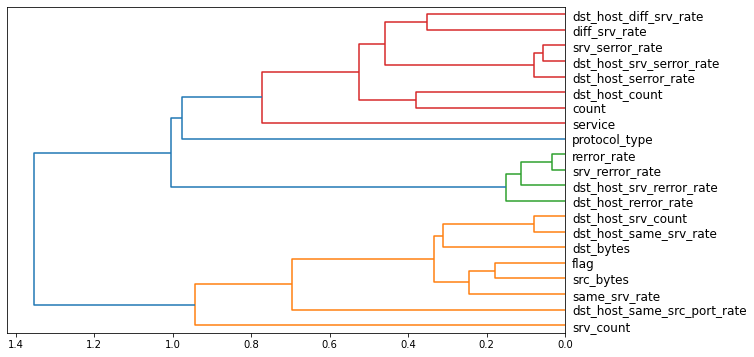```xs_final = xs_imp.drop(to_drop, axis=1)
valid_xs_final = valid_xs_imp.drop(to_drop, axis=1)
```

```m = rf(xs_final, y)
m_rmse(m, xs_final, y), m_rmse(m, valid_xs_final, valid_y)
```
`(0.9879073869900772, 0.9885696142244801)`

```p = valid_xs_final['count'].hist(density=True)
``````p = valid_xs_final['src_bytes'].hist(density=True)
```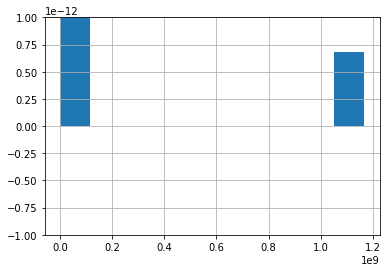```from sklearn.inspection import plot_partial_dependence
for target, target_id in name_to_id.items():
fig,ax = plt.subplots(figsize=(12, 4))
plt.title(label='Target name: {}'.format(target))
plot_partial_dependence(m, valid_xs_final, ['count','src_bytes'],
target=target_id, grid_resolution=20, ax=ax);
```
```/Users/home0/.pyenv/versions/3.7.8/lib/python3.7/site-packages/ipykernel_launcher.py:3: RuntimeWarning: More than 20 figures have been opened. Figures created through the pyplot interface (`matplotlib.pyplot.figure`) are retained until explicitly closed and may consume too much memory. (To control this warning, see the rcParam `figure.max_open_warning`).
This is separate from the ipykernel package so we can avoid doing imports until
/Users/home0/.pyenv/versions/3.7.8/lib/python3.7/site-packages/ipykernel_launcher.py:3: RuntimeWarning: More than 20 figures have been opened. Figures created through the pyplot interface (`matplotlib.pyplot.figure`) are retained until explicitly closed and may consume too much memory. (To control this warning, see the rcParam `figure.max_open_warning`).
This is separate from the ipykernel package so we can avoid doing imports until
/Users/home0/.pyenv/versions/3.7.8/lib/python3.7/site-packages/ipykernel_launcher.py:3: RuntimeWarning: More than 20 figures have been opened. Figures created through the pyplot interface (`matplotlib.pyplot.figure`) are retained until explicitly closed and may consume too much memory. (To control this warning, see the rcParam `figure.max_open_warning`).
This is separate from the ipykernel package so we can avoid doing imports until
```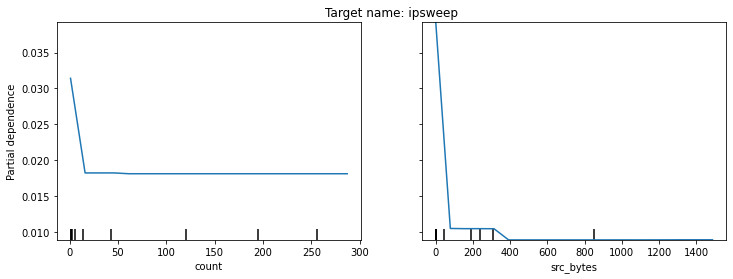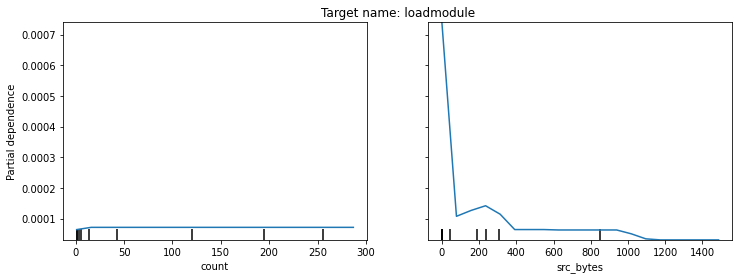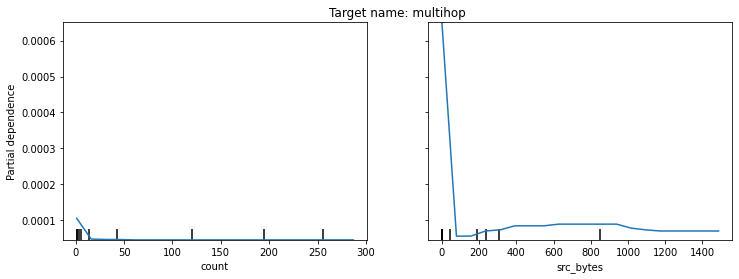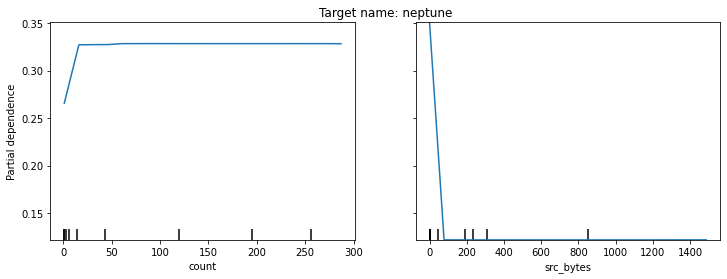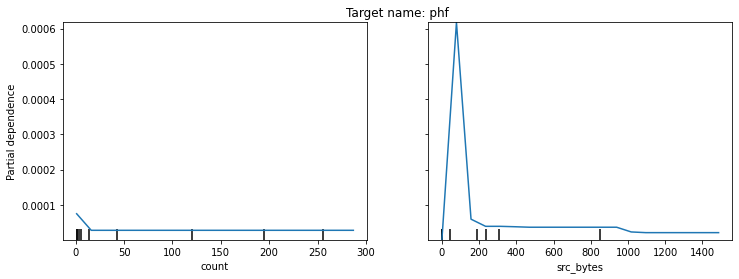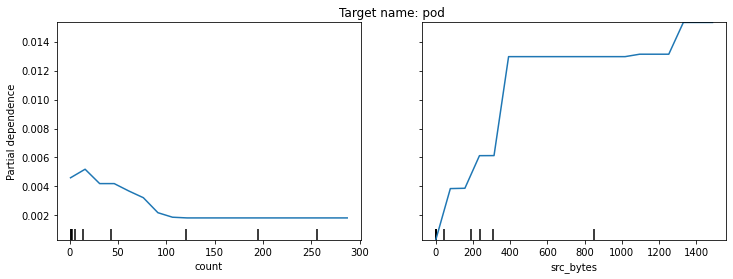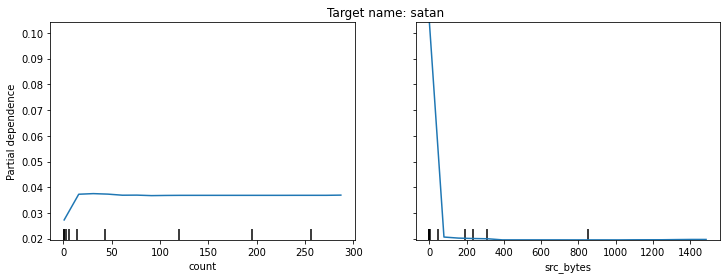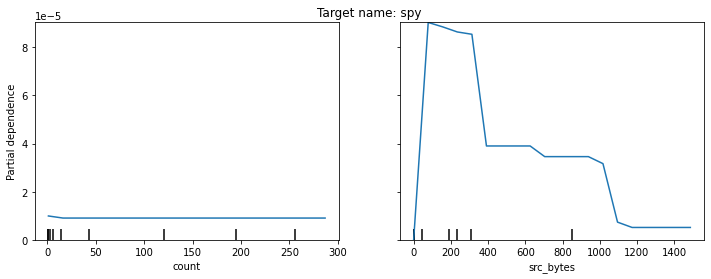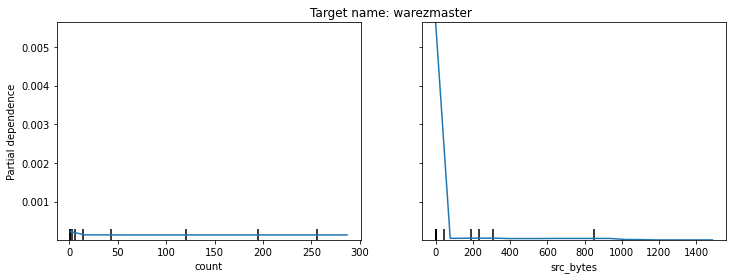## Examining Row-Level Feature Contributions

Examining the measures of contributions reveals how the random forest is determining the prediction for each instance in the training set. I had used `treeinterpreter` in the past but not `waterfall_chart`. These charts are nice, especially if shown in an array. We can, for example compare a correctly predicted row waterfall chart to an incorrectly predicted row outcome to reveal differences in that row that caused the false positive. Below that is shown.

```import warnings
warnings.simplefilter('ignore', FutureWarning)
```

```from treeinterpreter import treeinterpreter
from waterfall_chart import plot as waterfall
class TargetProfiler:
"""Profiler for a single target value of a multiple class dep_var"""
def __init__(self, est, valid_X, valid_y, dep_var,
target, name_to_id, n_components):
self.n_components = n_components
self.est = est
self.valid_X = valid_X
self.valid_y = valid_y
self.dep_var = dep_var
self.target = target
self.name_to_id = name_to_id
self.id_to_name = {idx: name for name, idx in self.name_to_id.items()}
prediction, bias, contributions = treeinterpreter.predict(
model=self.est, X=self.subset_xs.values
)
self.data = {
i: {
'preds': prediction[i],
'bias': bias[i],
'contribs': contributions[i]}
for i in range(prediction.shape)
}
self.pred_ans = [(row == row.max()).nonzero() for row in prediction]
self.confusion_arr = [id_to_name[ans] for ans in self.pred_ans]
self.hits = []
self.misses = []
for i, elem in enumerate(self.confusion_arr):
if elem==self.target:
self.hits.append(i)
else:
self.misses.append(i)

self.hit_contribs_profiles = []
self.miss_contribs_profiles = []
self.contrib_profile_summary = {
'hits': {},
'misses': {}
}
self._create_contrib_profiles()

def _create_contrib_profiles(self):
"""Creates the contribution objects and summary"""
for idx in self.hits:
self.hit_contribs_profiles.append(
self.get_top_n_contributions(idx=idx)
)

for idx in self.misses:
self.miss_contribs_profiles.append(
self.get_top_n_contributions(idx=idx)
)

for profile in self.hit_contribs_profiles:
if profile not in self.contrib_profile_summary['hits']:
self.contrib_profile_summary['hits'][profile] = 0

self.contrib_profile_summary['hits'][profile] += 1

self.contrib_profile_summary['hits'] = OrderedDict(
{k: v
for k, v
in sorted(
self.contrib_profile_summary['hits'].items(),
key=lambda item: item,
reverse=True)
}
)

for profile in self.miss_contribs_profiles:
if profile not in self.contrib_profile_summary['misses']:
self.contrib_profile_summary['misses'][profile] = 0
self.contrib_profile_summary['misses'][profile] += 1

self.contrib_profile_summary['misses'] = OrderedDict(
{k: v
for k, v
in sorted(
self.contrib_profile_summary['misses'].items(),
key=lambda item: item,
reverse=True)
}
)

def print_summary(self):
print('There are {} TP predictions on which contributions are measured'.format(len(self.hit_contribs_profiles)))
print('There are {} unique TP contribution profiles considering the top {} feature contributions'.format(len(set(self.hit_contribs_profiles)), self.n_components))
print('There are {} FP predictions on which contributions are measured'.format(len(self.miss_contribs_profiles)))
print('There are {} unique FP contribution profiles considering the top {} feature contributions'.format(len(set(self.miss_contribs_profiles)), self.n_components))

def get_top_n_contributions(self, idx, n=None):
if not n:
n = self.n_components
data = self.get_instance_contributions(idx=idx).tolist()
df = pd.DataFrame(data, index=self.subset_xs.columns)
df['abs'] = df.apply(abs)
df.sort_values(by='abs', inplace=True, ascending=False)
return tuple([(k,v) for k,v in df.iloc[:n].to_dict().items()])

def get_instance_contributions(self, idx):
"""
Finds the contribution array for the max probability
class for an instance row
"""
choice = name_to_id[self.confusion_arr[idx]]
choice_contributions = self.data[idx]['contribs'][:, choice]
return choice_contributions

def get_chart_config(self, idx, num_stds=0):
"""
Produces the variables necessary for the waterfall chart
"""
index=self.subset_xs.columns
data = self.get_instance_contributions(idx=idx)
threshold = self.get_instance_contributions(idx=idx).mean()+num_stds*self.get_instance_contributions(idx=idx).std()

Title =' '.join([
'instance id = {} in target="{}" predicted as "{}", (Pr={:.2f})',
'\nFiltering contributions < mu(contributions)+{}*sd(contributions)'
]) \
.format(idx, self.target, self.confusion_arr[idx], self.data[idx]['preds'].max(), num_stds)
sorted_value = True
net_label = 'Total'
rotation_value = 90
formatting = '{:,.3f}'

return index,data,rotation_value,threshold,sorted_value,net_label,formatting,Title
```

```profiler = TargetProfiler(
est=m, dep_var=dep_var, name_to_id=name_to_id,
valid_X=valid_xs_final, valid_y=valid_y, target='ipsweep',
n_components=1
)
```

```index,data,rotation_value,threshold,sorted_value,net_label,formatting,Title = \
profiler.get_chart_config(idx=0, num_stds=0)
waterfall(
index=index,
data=data,
rotation_value=rotation_value,
threshold=threshold,
sorted_value=sorted_value,
net_label=net_label,
formatting=formatting,
Title=Title
);
``````profiler.print_summary()
```
```There are 362 TP predictions on which contributions are measured
There are 17 unique TP contribution profiles considering the top 1 feature contributions
There are 6 FP predictions on which contributions are measured
There are 4 unique FP contribution profiles considering the top 1 feature contributions
```

```profiler.contrib_profile_summary
```
```{'hits': OrderedDict([((('protocol_type', 0.24009813957411152),), 261),
((('src_bytes', 0.3173731794970055),), 32),
((('dst_host_count', 0.3436304262560699),), 19),
((('src_bytes', 0.28486997315514756),), 15),
((('dst_host_count', 0.3361468403244449),), 10),
((('src_bytes', 0.31392163906054854),), 7),
((('dst_host_count', 0.33574879142352254),), 4),
((('dst_host_count', 0.23601070827400092),), 4),
((('dst_host_count', 0.34036114589529975),), 2),
((('dst_host_count', 0.21124475693546593),), 1),
((('dst_host_diff_srv_rate', 0.1749973119124059),), 1),
((('dst_host_count', 0.3448176195585743),), 1),
((('dst_host_diff_srv_rate', 0.21467104693847716),), 1),
((('src_bytes', 0.28053443494562785),), 1),
((('dst_host_diff_srv_rate', 0.17797272407802261),), 1),
((('src_bytes', 0.2895155847970092),), 1),
((('dst_host_diff_srv_rate', 0.21920854927635197),), 1)]),
'misses': OrderedDict([((('src_bytes', -0.3812063371755676),), 3),
((('dst_host_count', 0.3317236492280668),), 1),
((('src_bytes', -0.3449156395858148),), 1),
((('src_bytes', -0.368992935454482),), 1)])}```

### Summarizing the Contribution Analysis

Note: values may differ slightly in reproductions

The illustration above, including code, enables several useful lines of questioning that help improve understanding around why the model predicts what it predicts. In particular, it allows someone to examine the contributions for a specific target value (a label in a multi-label problem). We introduce the concept of a "contribution profile", which is the array of contribution metrics for every feature in the model as an estimate of the latent metric space that best describes the relationship between the independent and dependent variable in the Random Forest model.

For example in the case above it is to describe the predictions on `attack='back'`. We show a simplified case of defining the contribution profile as the single maximum contributing feature and its contribution value. In all cases of where the result was a true positive, the feature with largest contributions was `src_bytes`, and there were 11 different contribution values spanning from around 0.45 to 0.76. Looking at the object called `profiler.contrib_profile_summary` we note that 70 of the hits shared the same contribution of the `src_bytes` feature, which was a value of 0.7610 Additionally, we can visualize the contributions with a waterfall plot for examples of TPs and FPs, with various characteristics.

Below, we look at the waterfall chart of the first of 100 (out of 103 total) hit in the dataset where `attack=='back'`, which is found by specifying `idx=profiler.hitsidx=profiler.hits`. We can see that the contribution of `src_bytes == 0.761` and that means that it is one in the 70 common contribution profiles. Below that, we can look at the first of 3 misses in the dataset, which is specified by setting `idx=profiler.missesidx=profiler.misses`. The miss examined shows quite a different contribution profile, which can be noticed in the chart title where measure of model confidence is lower at 0.80 vs 0.97 in the hit. It can also be noticed because using the same `num_stds` filter yields a much more complicated profile with 14 features in it compared to the 4 feature profile of the hit. Concluding that we can eliminate quite a bit of the false positives in the prediction with a confidence based filter.

```index,data,rotation_value,threshold,sorted_value,net_label,formatting,Title = \
profiler.get_chart_config(idx=profiler.hits, num_stds=0)
waterfall(
index=index,
data=data,
rotation_value=rotation_value,
threshold=threshold,
sorted_value=sorted_value,
net_label=net_label,
formatting=formatting,
Title=Title
);
``````index,data,rotation_value,threshold,sorted_value,net_label,formatting,Title = \
profiler.get_chart_config(idx=profiler.misses, num_stds=0)
waterfall(
index=index,
data=data,
rotation_value=rotation_value,
threshold=threshold,
sorted_value=sorted_value,
net_label=net_label,
formatting=formatting,
Title=Title
);
```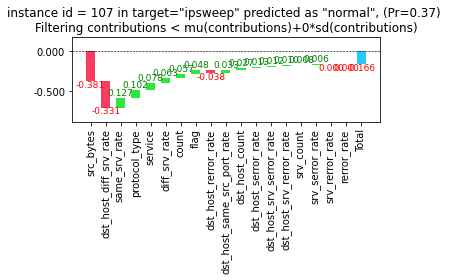## Examining Performance of Neural Networks

Bottom line, the basic neural network trained for a handful of epochs doesn't perform anywhere close to the immaculate performance of the sklearn CART Random Forest trained in under 10 seconds.

```from fastai.tabular.all import *
learn = tabular_learner(dls, layers=[500,250], n_out=len(y.unique()))
```

```learn.lr_find()
```
`SuggestedLRs(lr_min=0.010000000149011612, lr_steep=0.0012022644514217973)````learn.fit_one_cycle(20, 10E-1)
```
epoch train_loss valid_loss time
0 0.337709 1.144320 00:09
1 0.632443 0.747873 00:07
2 23.166491 22.490313 00:07
3 3.629263 19.744925 00:07
4 488.622559 127238760.000000 00:07
5 96.140236 620348.937500 00:07
6 11.745590 7905.200684 00:07
7 478.555511 150971.156250 00:08
8 101.396439 494598.312500 00:07
9 159.518036 3370265.000000 00:07
10 26.548840 151346.234375 00:07
11 24.579018 284696.968750 00:07
12 3.943957 10846.134766 00:07
13 2.275284 4242.146973 00:07
14 1.417892 2386.899902 00:07
15 1.279707 67.186714 00:07
16 1.260258 468.439819 00:07
17 1.256195 683.481873 00:07
18 1.254877 383.278656 00:07
19 1.256950 319.377411 00:07

```preds,targs = learn.get_preds()
chk = np.equal(preds.argmax(dim=1).unsqueeze(1), targs).squeeze(-1)
print('Accuracy:', chk.sum() / (chk.shape*1.0))
```
```Accuracy: tensor(0.5354)
```

## Conclusions

The KDD'99 dataset is particularly well suited to more traditional modeling techniques like decision trees and random forests. At least the basic attempt at fitting a neural network failed to produce an effective model, let alone a rockstart model such as that which was easily produced with the random forest. Likely, the KDD'99 dataset is massively cleaned and processed, which is why the decision trees work so well.

### Future Work

In the future, it would be interesting to examine the extent to which neural networks can be coaxed into performing better. Future work will likely involve finding a dataset that is a little more realistic.